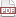### Tip 19: define persistent state variables

08 November 11. [link]PDF version

Part of a series of tips on POSIX and C. Start from the tip intro page, or get 21st Century C, the book based on this series.

level: intermediate
purpose: build more self-sufficient functions

A tip every other day on POSIX and C. Start from the tip intro page.

Static variables can have local scope. That is, you can have variables that exist only in one function, but when the function exits the variable retains its value. This is great for having an internal counter or a reusable scratch space.

Let's go with a traditional textbook example for this one: the Fibonacci sequence. Each element is the sum of the two prior elements.

#include <stdio.h>

long long int fibonacci(){
static long long int first = 1;
static long long int second = 1;
long long int out = first+second;
first=second;
second=out;
return out;
}

int main(){
for (int i=0; i< 50; i++)
printf("%Li\n", fibonacci());
}


Check out how insignficant main is. The fibonacci function is a little machine that runs itself; main just has to bump the function and it spits out another value. My language here isn't for cuteness: the function is a simple state machine, and static variables are the key trick for implementing state machines via C.

On to the tip: static variables are initialized when the program starts, before main, so you need to set its value to a constant.

//this fails: can't call gsl_vector_alloc() before main() starts
static gsl_vector *scratch = gsl_vector_alloc(20);


This is an annoyance (more next time), but easily solved with a macro to start at zero and allocate on first use:

#define Staticdef(type, var, initialization) \
static type var = 0; \
if (!(var)) var = (initialization);

//usage:
Staticdef(gsl_vector*, scratch, gsl_vector_alloc(20));


This works as long as we don't ever expect initialization to be zero (or in pointer-speak, NULL). If it is, it'll get re-initialized on the next go-round. Maybe that's OK anyway.